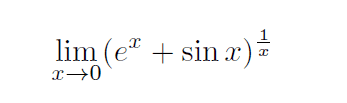# Small Limit Problem in L'Hospital's Rule Chapter

• Student4

#### Student4

Stuck on really starting this onerelevant info: Same chapter as L’Hospital’s Rule.

Any ideas?

Since this is in the same chapter as "L'Hopital's rule", it seems likely L'Hopital's rule will work!

To put it into that right form to use for L'Hopital's rule, take the logarithm of both sides:
$$ln(y)= ln((e^x+ sin(x))^{1/x})= \frac{ln(e^x+ sin(x)}{x}$$
Use L'Hopital's rule on that (both numerator and denominator go to 0), to find the limit of ln(y) then take the exponential to find the limit of y itself.